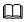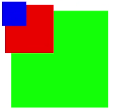svg说明：

• 该组件从API version 7开始支持。后续版本如有新增内容，则采用上角标单独标记该内容的起始版本。
• svg父组件或者svg组件需要定义宽高值，否则不进行绘制。

属性

id

string

-

width

<length>|<percentage>

-

height

<length>|<percentage>

-

x

<length>|<percentage>

-

y

<length>|<percentage>

viewBox

string

-

示例

<!-- xxx.hml -->
<div class="container">
<svg width="400" height="400">
<svg width="200" height="200" viewBox="0 0 100 100">
<rect x="10" y="10" width="80" height="80" fill="#00FF00"></rect>
</svg>
<rect x="10" y="10" width="80" height="80" fill="red" ></rect>
<svg x="0" y="0" width="200" height="200" viewBox="0 0 200 200">
<rect x="10" y="10" width="80" height="80" fill="red"></rect>
</svg>
<svg x="0" y="0" width="200" height="200" viewBox="0 0 400 400">
<rect x="10" y="10" width="80" height="80" fill="blue"></rect>
</svg>
</svg>
</div>
1
2
3
4
5
6
7
8
9
10
11
12
13
14
15